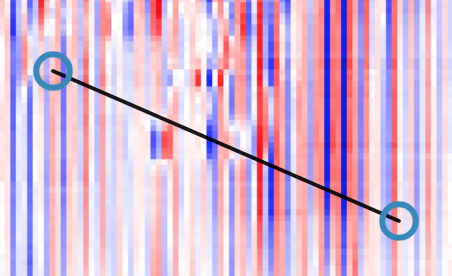# Collision detection

Hi, I have implemented a tool (which inherits from GestureTool) where user drags a line. User can then click on the line later to select it and edit it be dragging it around or moving its ends.
It looks like this:In order to calculate if the user has clicked on a line, I have implemented the following function which works well:

``````  ptOnLine(
pt_x: number,
pt_y: number,
ln_x1: number,
ln_y1: number,
ln_x2: number,
ln_y2: number,
slack: number = LINE_HIT_SLACK
) {
const d1 = Math.sqrt(Math.pow(pt_x - ln_x1, 2) + Math.pow(pt_y - ln_y1, 2));
const d2 = Math.sqrt(Math.pow(pt_x - ln_x2, 2) + Math.pow(pt_y - ln_y2, 2));
const d3 = Math.sqrt(
Math.pow(ln_x1 - ln_x2, 2) + Math.pow(ln_y1 - ln_y2, 2)
);
return d1 + d2 >= d3 - slack && d1 + d2 <= d3 + slack;
}
``````

In order to calculate if the user has clicked on a knob (circle - approximated as a square), I have implemented the following function which works well:

``````ptInBB(
pt_x: number,
pt_y: number,
bbc_x: number,
bbc_y: number,
): boolean {
return (
pt_x <= bbc_x + radius &&
pt_x >= bbc_x - radius &&
pt_y <= bbc_y + radius &&